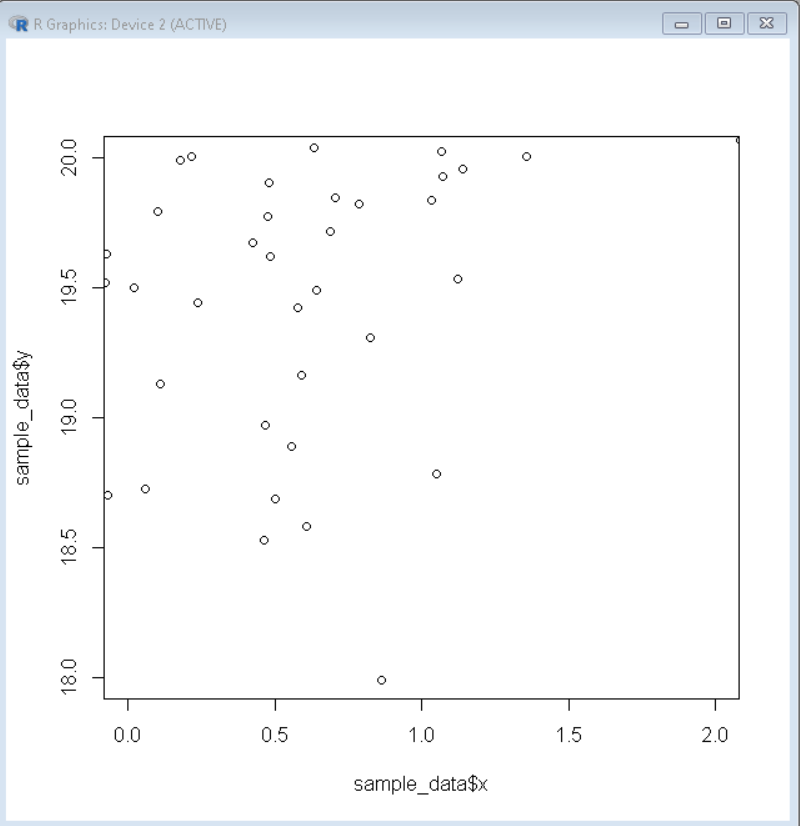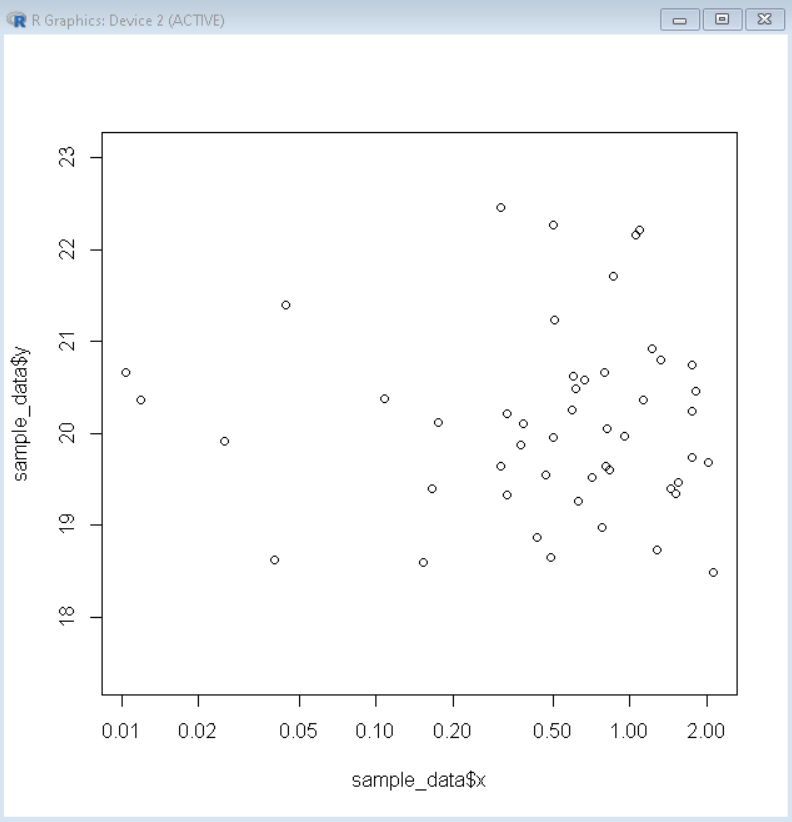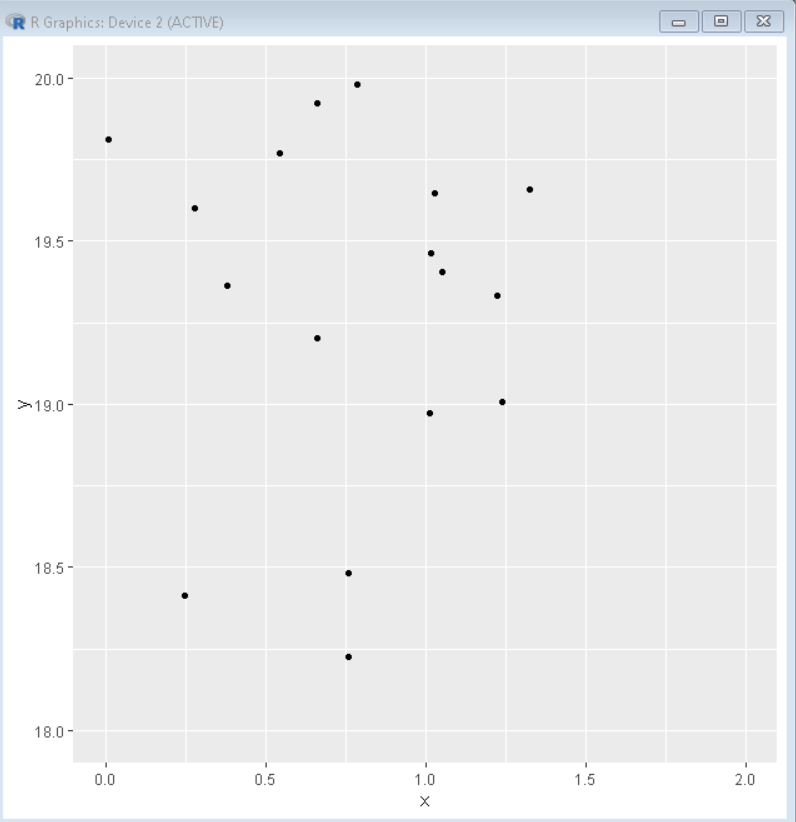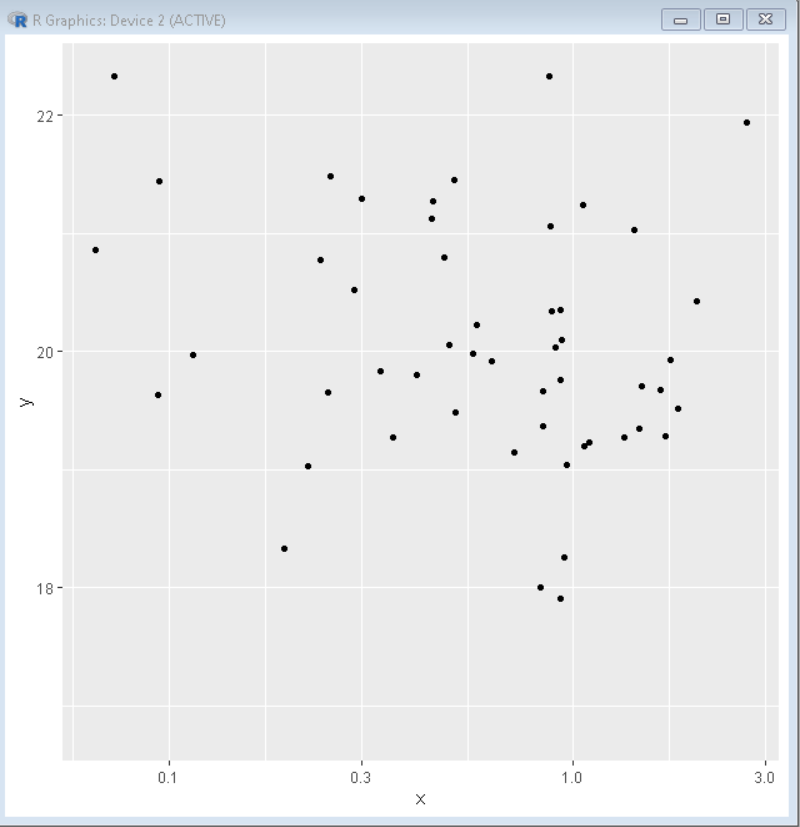GeeksforGeeks App
Open AppBrowser
Continue

# How to Change Axis Scales in R Plots?

In this article, we will learn how to change Axis Scales in the R Programming Language.

## Method 1: Change Axis Scales in Base R

To change the axis scales on a plot in base R Language, we can use the xlim() and ylim() functions. The xlim() and ylim() functions are convenience functions that set the limit of the x-axis and y-axis respectively. This function takes a vector as an argument which contains the values of lower axis limit and higher axis limit.

Syntax: plot( df\$xaxis, df\$yaxis, xlim, ylim)

where,

• df: determines the data frame in use.
• xaxis and yaxis: determine the axis variables for plotting.
• xlim: determines the vector that contains x-axis limits.
• ylim: determines the vector that contains y-axis limits.

Example: Basic example where plot axis limits are set from 0 to 2 for the x-axis and from 18 to 20 for the y-axis.

## R

 `# create sample data frame``sample_data <- ``data.frame``(x=``rnorm``(100),``                          ``y=``rnorm``(100)+20)`` ` `# create plot with custom axis scales``plot``(sample_data\$x, sample_data\$y, xlim=``c``(0,2),``     ``ylim=``c``(18,20))`

Output:Output

### Convert axis scales to log scale:

To convert the axis scale log scale in the base R plot, we use the log argument of the plot() function. The log argument converts the given axis into its log scale alternative. This helps us in visualizing the skew data frames.

Syntax: plot( df\$xaxis, df\$yaxis, log)

where,

• df: determines the data frame in use.
• xaxis and yaxis: determine the axis variables for plotting.
• log: determines the axis which has to be converted in log scale.

Example: Basic example where the x-axis has been converted to its log scale alternative.

## R

 `# create sample data frame``sample_data <- ``data.frame``(x=``rnorm``(100),``                          ``y=``rnorm``(100)+20)`` ` `# create plot with log x-axis scale``plot``(sample_data\$x, sample_data\$y, log=``'x'``)`

Output:Output

## Method 2: Change Axis Scales in ggplot2

To change the axis scales on a plot made using the ggplot2 package in the R Language, we can use the xlim() and ylim() functions. These functions can be used along with the ggplot() function by adding them using plus(+) symbol The xlim() and ylim() functions are convenience functions that set the limit of the x-axis and y-axis respectively. This function takes a vector as an argument which contains the values of lower axis limit and higher axis limit.

Syntax:

ggplot() + xlim() +ylim()

where,

xlim(): takes two values as input that are lower x-axis limit and higher x-axis limit.

ylim(): takes two values as input that are lower y-axis limit and higher y-axis limit.

Example:

Here, is a basic example of a ggplot2 plot where plot axis limits are set from 0 to 2 for the x-axis and from 18 to 20 for the y-axis.

## R

 `# load library ggplot2``library``(ggplot2)`` ` `# create sample data frame``sample_data <- ``data.frame``(x=``rnorm``(100),``                          ``y=``rnorm``(100)+20)`` ` `# create plot with custom axis scales``ggplot``(sample_data, ``aes``(x=x, y=y))+``        ``geom_point``()+``        ``xlim``(0,2)+``        ``ylim``(18,20)`

Output:Output

### Convert axis scales to log scale:

To convert the axis scale log scale in the R plot made using the ggplot2 package, we use the scale_y_continuous() and scale_y_continuous() functions along with the trans argument for the x-axis and the y-axis transformation respectively. The trans argument takes a logarithmic identifier as an argument and then converts the axis into the given log scale alternative. This helps us in visualizing the skew data frames.

Syntax: plot + scale_x_continuous( trans ) + scale_y_continuous( trans )

where, trans: determines the exact log scale for transformation

Example: Basic example of a ggplot2 plot where the x-axis has been converted to its log scale alternative.

## R

 `# load library ggplot2``library``(ggplot2)`` ` `# create sample data frame``sample_data <- ``data.frame``(x=``rnorm``(100),``                          ``y=``rnorm``(100)+20)`` ` `# create plot with log x-axis sclae``ggplot``(sample_data, ``aes``(x=x, y=y))+``        ``geom_point``()+``        ``scale_x_continuous``( trans= ``'log10'``)`

Output:Output

My Personal Notes arrow_drop_up